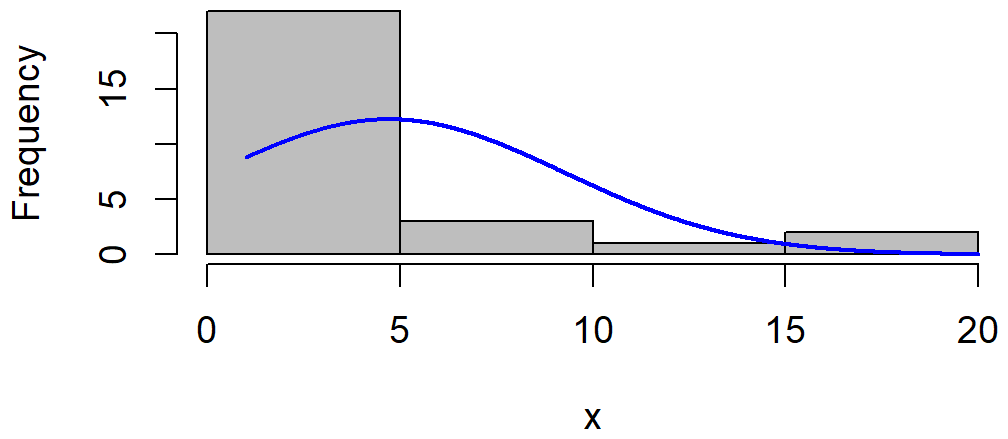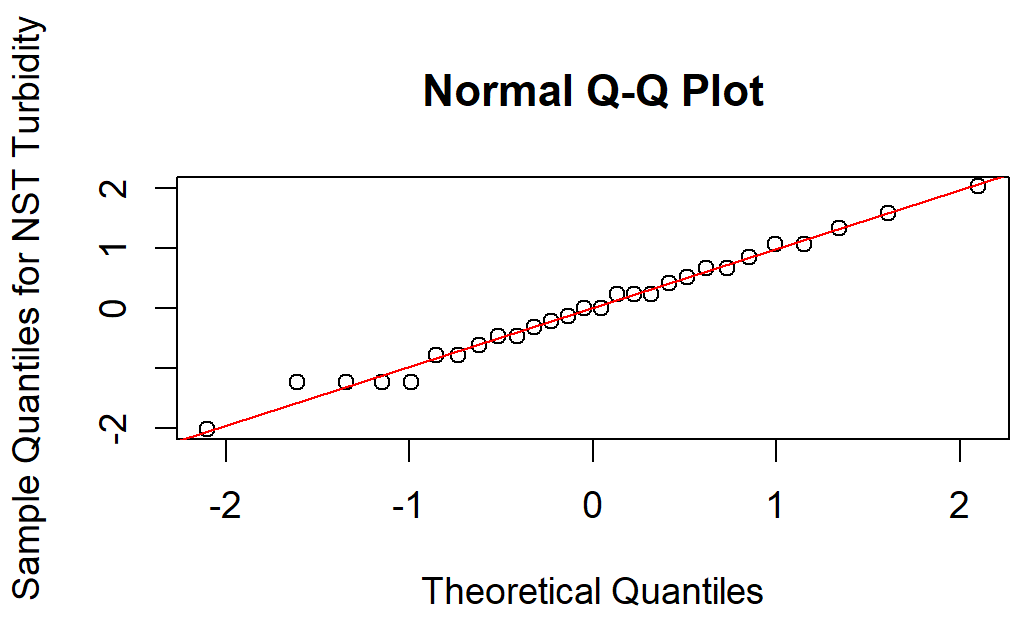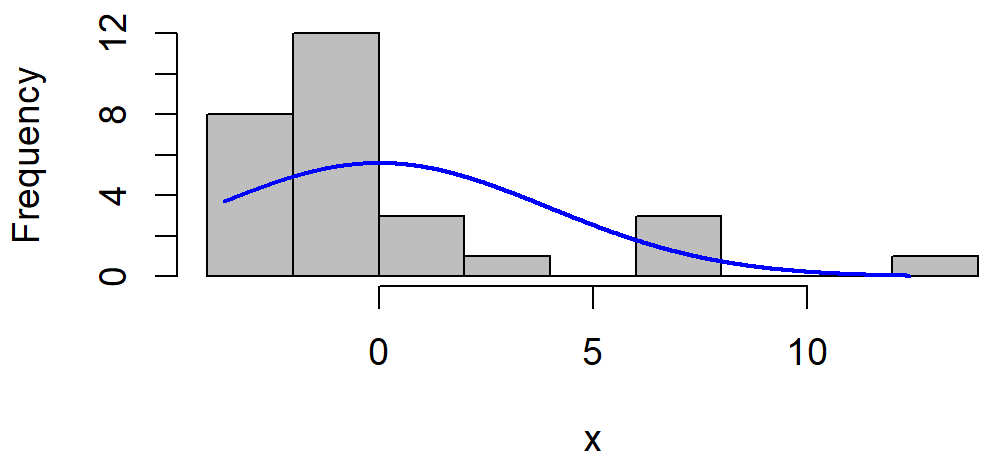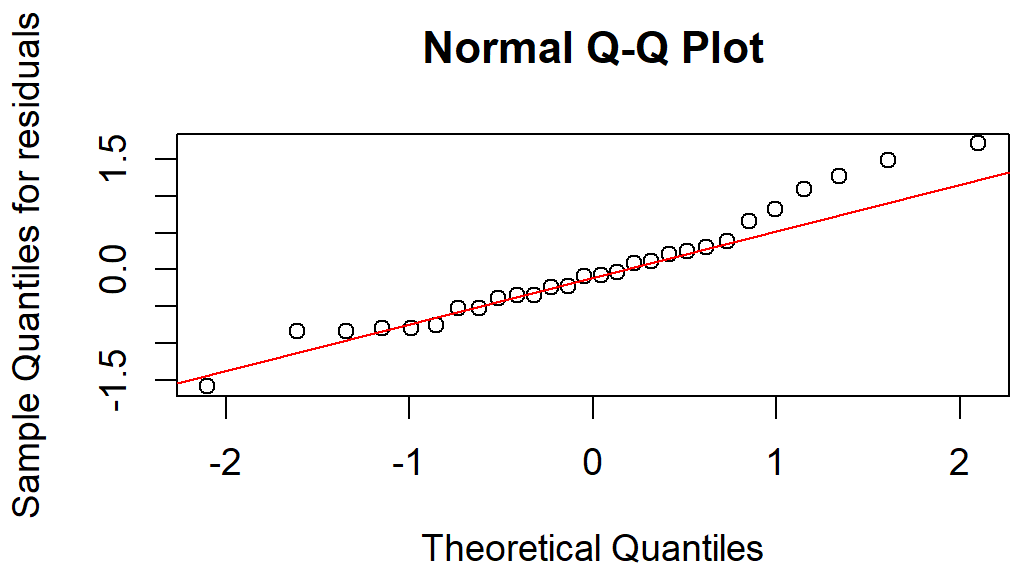﻿ R Handbook: Normal Scores Transformation## Summary and Analysis of Extension Program Evaluation in R

Salvatore S. Mangiafico

# Normal Scores Transformation

The previous chapter addressed some commonly used transformations.  Another transformation technique is normal scores transformation, or inverse normal transformation.

Normal scores transformation is useful to coerce a variable to a standard normal distribution.

The blom function in the rcompanion package can transform a single variable with a few different normal scores transformation methods.  The default method used by this function is the Elfving method, with options for Blom, van der Waerden, Tukey, and rankit methods.  It can also perform z score transformation and scaling a variable to a specified range.

This chapter will address the turbidity data used in the previous chapter.

### Packages used in this chapter

The packages used in this chapter include:

•  rcompanion

The following commands will install these packages if they are not already installed:

if(!require(rcompanion)){install.packages("rcompanion")}

### Example of normal scores transformation

In this hypothetical data set, the distribution for turbidity is quite skewed.

Input =("
Location Turbidity
a        1.0
a        1.2
a        1.1
a        1.1
a        2.4
a        2.2
a        2.6
a        4.1
a        5.0
a       10.0
b        4.0
b        4.1
b        4.2
b        4.1
b        5.1
b        4.5
b        5.0
b       15.2
b       10.0
b       20.0
c        1.1
c        1.1
c        1.2
c        1.6
c        2.2
c        3.0
c        4.0
c       10.5
")

library(rcompanion)

plotNormalHistogram(Data\$Turbidity)qqnorm(Data\$Turbidity,
ylab="Sample Quantiles for Turbidity")

qqline(Data\$Turbidity, col="red")#### Normal scores transformation

Here, the normal scores transformation results in a variable that is fairly close to a normal distribution, with a mean of approximately zero and standard deviation of approximately one.

library(rcompanion)

Data\$TurbidityNST = blom(Data\$Turbidity)

plotNormalHistogram(Data\$TurbidityNST)qqnorm(Data\$TurbidityNST,
ylab="Sample Quantiles for NST Turbidity")

qqline(Data\$TurbidityNST, col="red")mean(Data\$TurbidityNST)

 0.004098743

sd(Data\$TurbidityNST)

 0.9635998

#### Attempt ANOVA on un-transformed data

As seen in the last chapter, the residuals from the analysis deviate from the normal distribution, perhaps enough to make the analysis invalid.  The plot of the residuals vs. the fitted values shows that the residuals are somewhat heteroscedastic, though not terribly so.  The boxplot suggests that the data within some groups are relatively skewed.

boxplot(Turbidity ~ Location,
data = Data,
ylab="Turbidity",
xlab="Location")model = lm(Turbidity ~ Location,
data=Data)

library(car)

Anova(model, type="II")

Anova Table (Type II tests)

Sum Sq Df F value  Pr(>F)
Location  132.63  2  3.8651 0.03447 *
Residuals 428.95 25

x = (residuals(model))

library(rcompanion)

plotNormalHistogram(x)qqnorm(residuals(model),
ylab="Sample Quantiles for residuals")

qqline(residuals(model),
col="red")plot(fitted(model),
residuals(model))#### ANOVA with normal scores transformed data

In this case, after transformation, the residuals from the ANOVA are closer to a normal distribution—although not perfectly—, making the F-test more appropriate.  The plot of the residuals vs. the fitted values shows that the residuals are reasonably homoscedastic.

boxplot(TurbidityNST ~ Location,

data = Data,

ylab="NST Turbidity",

xlab="Location")model = lm(TurbidityNST ~ Location, data=Data)

library(car)

Anova(model, type="II")

Anova Table (Type II tests)

Response: TurbidityNST
Sum Sq Df F value   Pr(>F)
Location   8.7627  2  6.7167 0.004628 **
Residuals 16.3075 25

x = residuals(model)

library(rcompanion)

plotNormalHistogram(x)qqnorm(residuals(model),
ylab="Sample Quantiles for residuals")

qqline(residuals(model),
col="red")plot(fitted(model),
residuals(model))### Conclusions

In this case, the normal scores transformation on the dependent variable resulted in a model whose residuals met the assumptions of normal distribution and homoscedasticity fairly well.  This may not be the case in all situations.